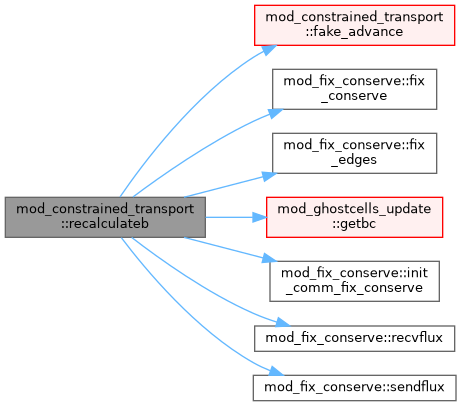MPI-AMRVAC  3.0 The MPI - Adaptive Mesh Refinement - Versatile Advection Code
mod_constrained_transport Module Reference

## Functions/Subroutines

subroutine recalculateb
re-calculate the magnetic field from the vector potential in a completely divergency free way More...

fake advance a step to calculate magnetic field More...

subroutine fake_update (ixIL, s, fC, fE, dxD)
fake update magnetic field from vector potential More...

subroutine b_from_vector_potentiala (ixIsL, ixIL, ixOL, ws, x, A)
calculate magnetic field from vector potential A at cell edges More...

subroutine reconstruct (ixIL, ixCL, idir, q, qL, qR)
Reconstruct scalar q within ixO^L to 1/2 dx in direction idir Return both left and right reconstructed values. More...

## ◆ b_from_vector_potentiala()

 subroutine mod_constrained_transport::b_from_vector_potentiala ( integer, intent(in) ixIs, integer, intent(in) L, integer, intent(in) ixI, L, integer, intent(in) ixO, L, double precision, dimension(ixis^s,1:nws), intent(inout) ws, double precision, dimension(ixi^s,1:ndim), intent(in) x, double precision, dimension(ixis^s,1:3), intent(inout) A )

calculate magnetic field from vector potential A at cell edges

Definition at line 103 of file mod_constrained_transport.t.

 subroutine mod_constrained_transport::fake_advance ( integer igrid, integer idim, integer LIM, type(state) s )

fake advance a step to calculate magnetic field

Definition at line 50 of file mod_constrained_transport.t.

Here is the call graph for this function:## ◆ fake_update()

 subroutine mod_constrained_transport::fake_update ( integer ixI, integer L, type(state) s, double precision, dimension(ixi^s,1:nwflux,1:ndim) fC, double precision, dimension(ixi^s,7-2*ndim:3) fE, double precision dx, double precision D )

fake update magnetic field from vector potential

Definition at line 71 of file mod_constrained_transport.t.

Here is the call graph for this function:## ◆ recalculateb()

 subroutine mod_constrained_transport::recalculateb

re-calculate the magnetic field from the vector potential in a completely divergency free way

Definition at line 8 of file mod_constrained_transport.t.

Here is the call graph for this function:## ◆ reconstruct()

 subroutine mod_constrained_transport::reconstruct ( integer, intent(in) ixI, integer, intent(in) L, integer, intent(in) ixC, L, integer, intent(in) idir, double precision, dimension(ixi^s), intent(in) q, double precision, dimension(ixi^s), intent(out) qL, double precision, dimension(ixi^s), intent(out) qR )

Reconstruct scalar q within ixO^L to 1/2 dx in direction idir Return both left and right reconstructed values.

Definition at line 177 of file mod_constrained_transport.t.

Here is the call graph for this function: